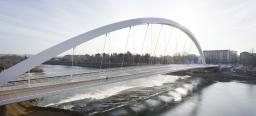# A bridge

A bridge over a river has the shape of the arc with bases of the bridge at the river's edge. At the center of the river, the bridge is 10 feet above the water. At 27 feet from the edge of the river, the bridge is 9 feet above the water. How wide is the river?

Correct result:

a =  80 ft

#### Solution:

Our quadratic equation calculator calculates it.We would be pleased if you find an error in the word problem, spelling mistakes, or inaccuracies and send it to us. Thank you!Dr Math
THE BRIDGE OVER THE RIVERDr Math
r = radius of circle of arc

x = distance between center of circle and water level (center of the circle is under ground)

y = distance in horiznotal of point on circle which is 9 ft above the waterTips to related online calculators
Looking for help with calculating roots of a quadratic equation?
Do you have a system of equations and looking for calculator system of linear equations?
Pythagorean theorem is the base for the right triangle calculator.

#### You need to know the following knowledge to solve this word math problem:

We encourage you to watch this tutorial video on this math problem:

## Next similar math problems:

• MO SK/CZ Z9–I–3John had the ball that rolled into the pool and it swam in the water. Its highest point was 2 cm above the surface. Diameter of circle that marked the water level on the surface of the ball was 8 cm. Determine the diameter of John ball.
• Find the 5Find the equation of the circle with center at (1,20), which touches the line 8x+5y-19=0
• Two chordsCalculate the length of chord AB and perpendicular chord BC to circle if AB is 4 cm from the center of the circle and BC 8 cm from the center of the circle.
• Secret treasureScouts have a tent in the shape of a regular quadrilateral pyramid with a side of the base 4 m and a height of 3 m. Determine the radius r (and height h) of the container so that they can hide the largest possible treasure.
• Points on circleIn the Cartesian coordinate system with the origin O is a sketched circle k /O; r=2 cm/. Write all the points that lie on a circle k and whose coordinates are integers. Write all the points that lie on the circle I / O; r=5 cm / and whose coordinates are
• Circle and squareAn ABCD square with a side length of 100 mm is given. Calculate the radius of the circle that passes through the vertices B, C and the center of the side AD.
• ChordIn a circle with radius r=60 cm is chord 4× longer than its distance from the center. What is the length of the chord?
• Quarter circleWhat is the radius of a circle inscribed in the quarter circle with a radius of 100 cm?
• Right triangleLegs of the right triangle are in the ratio a:b = 2:8. The hypotenuse has a length of 87 cm. Calculate the perimeter and area of the triangle.
• Circle chordCalculate the length of the chord of the circle with radius r = 10 cm, length of which is equal to the distance from the center of the circle.
• 9-gon pyramidCalculate the volume and the surface of a nine-sided pyramid, the base of which can be inscribed with a circle with radius ρ = 7.2 cm and whose side edge s = 10.9 cm.
• Sides of right angled triangleOne leg is 1 m shorter than the hypotenuse, and the second leg is 2 m shorter than the hypotenuse. Find the lengths of all sides of the right-angled triangle.
• ArcCalculate span of the arc, which is part of a circle with diameter d = 20 m and its height is 6 m.
• Euclid theoremsCalculate the sides of a right triangle if leg a = 6 cm and a section of the hypotenuse, which is located adjacent the second leg b is 5cm.
• Triangular pyramidA regular tetrahedron is a triangular pyramid whose base and walls are identical equilateral triangles. Calculate the height of this body if the edge length is a = 8 cm
• Top of the towerThe top of the tower has the shape of a regular hexagonal pyramid. The base edge has a length of 1.2 m, the pyramid height is 1.6 m. How many square meters of sheet metal is needed to cover the top of the tower if 15% extra sheet metal is needed for joint
• An equilateralAn equilateral triangle is inscribed in a square of side 1 unit long so that it has one common vertex with the square. What is the area of the inscribed triangle?